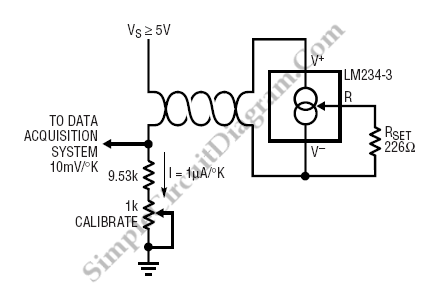# Temperature Sensor Current Loop Transmitter

LM134 makes a good temperature sensor because it has a highly linear output characteristic. Because it is a current output device and is therefore not affected by long wire runs, LM134 is particularly useful in remote sensing applications. LM134 is easy to calibrate. It has good long term stability and can be interfaced directly with most data acquisition system, so it can eliminate the expensive pre-amplifiers which is required for thermocouples and platinum sensors. Figure below shows a typical temperature sensor application.The output of 1mA/’K will be produced if the LM134 operating current at 25’C is set at 298mA by the 226W resistor. The current flows through the twisted pair sensors leads to the 10K terminator resistor, this will make the current output converted to a voltage of 10mV/’K referred to ground. The magnitude of voltage across the 10K resistor at 25’C will be 2.98V, with a slope of 10mV/’C. Subtract the a constant 2.73V in software is the simplest way to convert this signal to a centigrade scale.

The resistor (RSET) which is used to set the operating current of the LM134 in temperature sensing applications should have good long term stability and low temperature coefficient. The slope of the temperature sensor is changed 1 % by a 30ppm/’C drifted in the resistor. This with assumption that the resistor is at the same temperature as the sensor, which is usually the case is to prevent errors due to wire resistance since the resistor should be located physically close to the LM134. A 1’C temperature error is created by a long term shift of 0.3% in the resistor. Stable resistors must be used for best long term performance since the long term drift of the LM134 is typically much better than this.

As mentioned above, LM134 is easy to calibrate. Referring to the figure, we can calibrate LM134 by trimming the termination resistor. Theoretically, this will trim both zero and slope simultaneously for Centigrade and Fahrenheit applications. Just like the actual output, the initial errors in LM134 are directly proportional to absolute temperature. This will make the the sensor is allowed to be trimmed at any temperature and have the slope error be corrected at the same time. After this single trim is completed, residual slope error is typically less than 1 %.